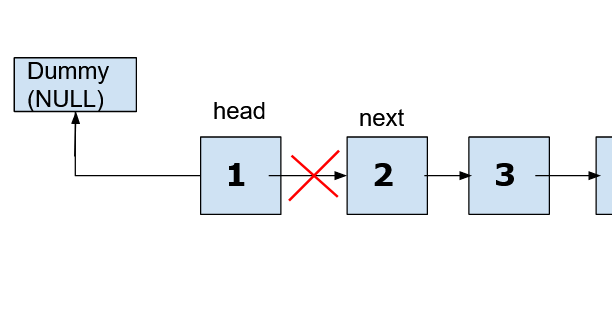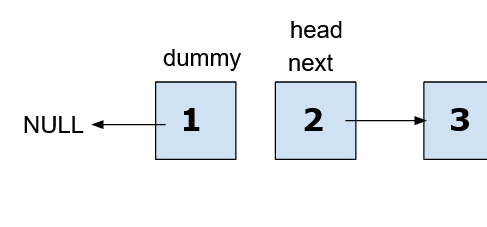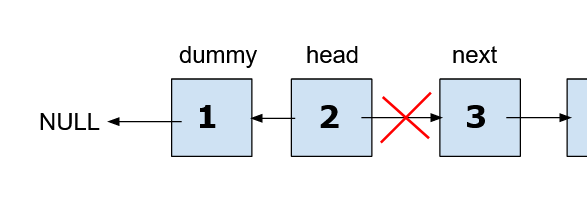# Reverse a Linked List

Problem Statement: Given the head of a singly linked list, write a program to reverse the linked list, and return the head pointer to the reversed list.

Examples:

```Input Format:
This means the given linked list is 3->6->8->10 with head pointer at node 3.

Result:
Output = [10,6,8,3]
This means, after reversal, the list should be 10->6->8->3 with the head pointer at node 10.```

Explanation

This is how a linked list looks for a given input. the head is a reference that points to the initial or starting node of the list. To reverse it, we need to invert the linking between nodes. That is, node D should point to node C, node C to node B, and node B to node A. Then the head points to node D and node A has NULL.

```Input Format:
This means the given linked list is empty.

Result:
Output = []

Explanation:
The linked list is empty. That is, no nodes are present in the list. Thus, even reversing will give the list as empty.```

Solution

Disclaimer: Don’t jump directly to the solution, try it out yourself first.

## Reverse Linked List: Iterative

We will use three-pointers to traverse through the entire list and interchange links between nodes. One pointer to keep track of the current node in the list. The second one is to keep track of the previous node to the current node and change links. Lastly, a pointer to keep track of nodes in front of current nodes.

STEP 1:

• currrent_p is a pointer to keep track of current nodes. Set it to head.
• prev_p is a pointer to keep track of previous nodes. Set it to NULL.
• next_p is a pointer to keep track of the next nodes.

STEP 2:

• Set next_p to point next node to node pointed by current_p.
• Change link between nodes pointed by current_p and prev_p.
• Update prev_p to current_p and current_p pointer to next_p.

Perform STEP 2 until current_p reaches the end of the list.

STEP 3:

Set head as prev_p. This makes the head point at the last node.

Dry Run:

At beginning,

As per this example, current_p points node with 3 and prev_p points to NULL

Now, we start iterating throughout the list until current_p has a value equal to NULL. Set next_p to the next node of the current_p pointed node present in the list.

Since the next node to the current_p pointed node is B. Therefore next_p points to node B.

Now, interchange linking between nodes pointed by prev_p and next_p,i.e, node A and NULL. Then, prev_p moves to node A, current_p moves to B, and next_p to C.

Thus, at the first iteration, we pointed node A from node B to NULL.

In the second iteration, we point node B from node C to node A. Move next_p to node D, current_p to node C, and prev_p to node A. This is performed in the same steps.

In the third iteration, we point node C from node D to node B. Move next_p out of the list, current_p to node D, and prev_p to node C.

In the fourth iteration, we point node D from NULL to node C. Move current_p out of the list and prev_p to node D.

Since the current_p value is equal to NULL so iteration stops. We set head as prev_p.

Hence, we achieved our reversed list.

Code:

## C++ Code

``````/**
* Definition for singly-linked list.
* struct ListNode {
*     int val;
*     ListNode *next;
*     ListNode() : val(0), next(nullptr) {}
*     ListNode(int x) : val(x), next(nullptr) {}
*     ListNode(int x, ListNode *next) : val(x), next(next) {}
* };
*/

class Solution {
public:

ListNode* reverseList(ListNode* head) {

//step 1
ListNode* prev_p = NULL;
ListNode* current_p = head;
ListNode* next_p;

//step 2
while(current_p) {

next_p = current_p->next;
current_p->next = prev_p;

prev_p = current_p;
current_p = next_p;
}

head = prev_p; //step 3
}
};``````

Time Complexity:

Since we are iterating only once through the list and achieving reversed list. Thus, the time complexity is O(N) where N is the number of nodes present in the list.

Space Complexity:

To perform given tasks, no external spaces are used except three-pointers. So, space complexity is O(1).

## Reverse a Linked List: Recursive

Intuition: This approach is very similar to the above 3 pointer approach. In the process of reversing, the base operation is manipulating the pointers of each node and at the end, the original head should be pointing towards NULL and the original last node should be the ‘head’ of the reversed Linked List.

Approach:

• In this type of scenario, we first take a dummy node that will be assigned to NULL.
• Then we take a next pointer which will be initialized to head->next and in future iterations, next will again be set to head->next
• Now coming to changes on the head node, as we have set the dummy node as NULL and next to head->next, we can now update the next pointer of the head to the dummy node.
•• Before moving to the next iteration dummy is set to head and then the head is set to the next node.
•• Now coming to the next iteration: We’ll follow a similar process to set next as head->next and update head->next = dummy, a dummy set to head, and head set to next
•• These iterations will keep going while the head of the original Linked List is not NULL, i.e. we’ll reach the end of the original Linked List and the Linked List has been reversed.

Code:

## C++ Code

``````/**
* Definition for singly-linked list.
* struct ListNode {
*     int val;
*     ListNode *next;
*     ListNode() : val(0), next(nullptr) {}
*     ListNode(int x) : val(x), next(nullptr) {}
*     ListNode(int x, ListNode *next) : val(x), next(next) {}
* };
*/

class Solution {
public:
ListNode* reverseList(ListNode* head) {
ListNode *newHead = NULL;
while (head != NULL) {
ListNode *next = head->next;
}
}
};
``````

## Java Code

``````/**
* Definition for singly-linked list.
* public class ListNode {
*     int val;
*     ListNode next;
*     ListNode() {}
*     ListNode(int val) { this.val = val; }
*     ListNode(int val, ListNode next) { this.val = val; this.next = next; }
* }
*/

class Solution {
public ListNode reverseList(ListNode head) {
ListNode newHead = null;
while (head != null) {
ListNode next = head.next;
}
}
}``````

Solution 3: Using Recursion

We traverse to the end of the list recursively.

As we reach the end of the list, we make the end node the head. Then receive previous nodes and make them connected to the last one.

At last, we link the second node to the head and the first node to NULL. We return to our new head.

Code:

## C++ Code

``````/**
* Definition for singly-linked list.
* struct ListNode {
*     int val;
*     ListNode *next;
*     ListNode() : val(0), next(nullptr) {}
*     ListNode(int x) : val(x), next(nullptr) {}
*     ListNode(int x, ListNode *next) : val(x), next(next) {}
* };
*/

class Solution {
public:

ListNode* reverseList(ListNode* &head) {

ListNode* nnode = reverseList(head->next);
return nnode;
}
};
``````

Dry Run:

Once we call a function to reach the end of the list, we get a reversed list except for the first node and second node.

Then we link node B to node A and node A to NULL.

Time Complexity:

We move to the end of the list and achieve our reversed list. Thus, the time complexity is O(N) where N represents the number of nodes.

Space Complexity:

Apart from recursion using stack space, no external storage is used. Thus, space complexity is O(1).

Special thanks to Dewanshi Paul and Aditya Shahare for contributing to this article on takeUforward. If you also wish to share your knowledge with the takeUforward fam, please check out this article.